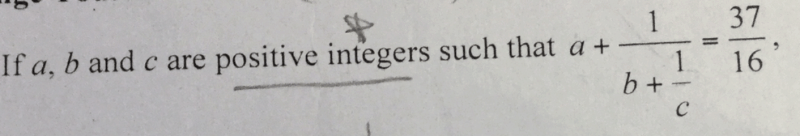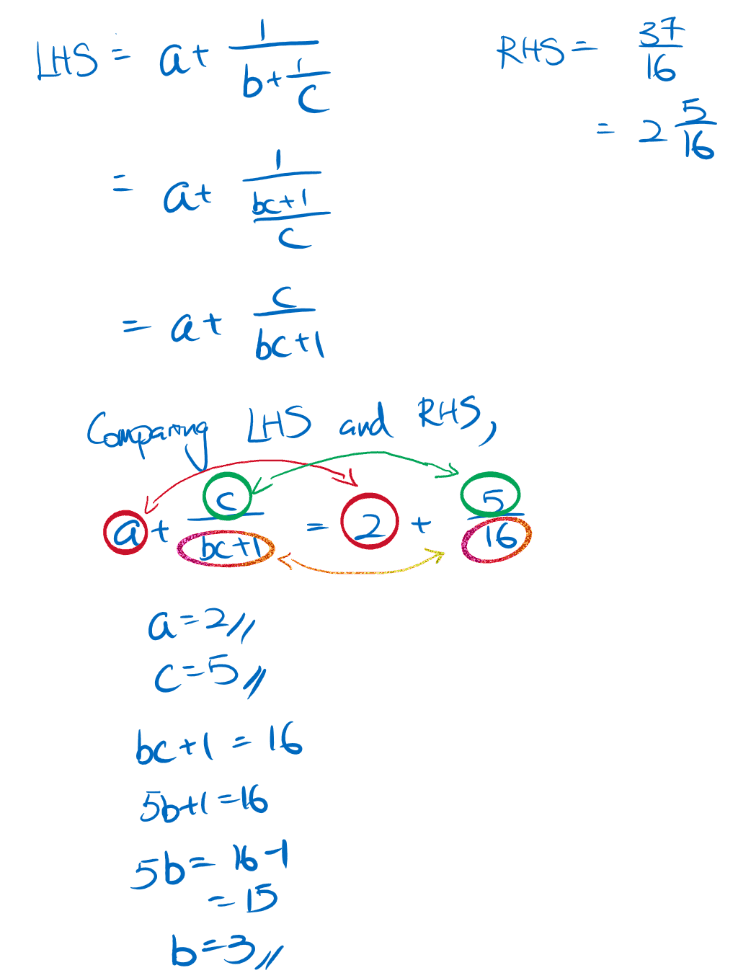# Question0 Replies 1 Like ✔Accepted Answer

Firstly, we note that b + 1/c must be greater than 1, so 1/ (b + 1/c) will be smaller than 1. Hence, a must “absorb” the whole number part of 37/16.

In other words, a = 37 // 16 = 2

This leaves us with 5/16 = 1/ (b + 1/c), or b + 1/c = 16/5. Using the same argument, we get:

b = 16//5 = 3 and 1/c = 1/5 (c = 5).

Therefore, a = 2, b = 3, c = 5.

0 Replies 0 Likes